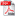# NUMERICAL AND EXPERIMENTAL ANALYSIS OF A HEAT EXCHANGER FOR THERMOELECTRIC REFRIGERATION

Autor/autori: *Alexandru-Adrian Prodan, *Mihai-Marius Prodan, Marius-Vasile Atanasiu

Abstract: As in recent years the development of numerical models has changed the way of solving various and complex problems, it is important to understand the capabilities and limitations of this powerful tool. Here, this is done by comparing the output of an in-house program, which numerically solves the heat conduction equation using the finite difference method, and a thermographic set of images of the spatial domain obtained in laboratory. The program was developed in MATLAB and uses the Forward Euler discretization scheme in time and Crank-Nicolson in space. The mesh resembles the geometry of a rectangular plate made of aluminium. The paper presents and explains the discrepancies between the two sets of results, quantifying the errors of the generated output. After verification, the computational tool is used to simulate the heat propagation on the cold plate of a thermoelectric refrigerator. In order to validate the simulation, the results are as well evaluated against the thermographic inspection of the computational domain. These techniques could also be used in the work of improving thermoelectric refrigeration systems

Keywords: discretization, heat conduction equation, thermoelectric refrigerationDOWNLOAD PDF